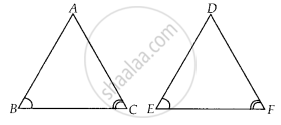# In triangles ABC and DEF, ∠B = ∠E, ∠F = ∠C and AB = 3 DE. Then, the two triangles are ______. - Mathematics

MCQ
Fill in the Blanks

In triangles ABC and DEF, ∠B = ∠E, ∠F = ∠C and AB = 3DE. Then, the two triangles are ______.

#### Options

• congruent but not similar

• similar but not congruent

• neither congruent nor similar

• congruent as well as similar

#### Solution

In triangles ABC and DEF, ∠B = ∠E, ∠F = ∠C and AB = 3DE. Then, the two triangles are similar but not congruent.

Explanation:

In ∆ABC and ∆DEF, ∠B = ∠E,

∠F = ∠C and AB = 3DEWe know that, if in two triangles corresponding two angles are same, then they are similar by AA similarity criterion.

Since, AB ≠ DE

Therefore ∆ABC and ∆DEF are not congruent.

Concept: Similarity of Triangles
Is there an error in this question or solution?

#### APPEARS IN

NCERT Mathematics Exemplar Class 10
Chapter 6 Triangles
Exercise 6.1 | Q 7 | Page 62
Share